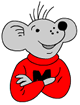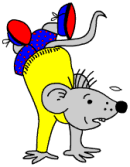## Free Y5 maths worksheets: 3-D shapeTwo very similar worksheets looking at the properties of 3-D shapes. Counting the number of faces, edges and vertices can be quite tricky and it is easier to do if there is a shape to look at and pick up.

Tetrahedron and octahedron are two new shapes to learn about. A tetrahedron has four faces whilst an octahedron has 8.

Free Y5 maths worksheet: 3-D shape (pg 2)

## Free Y4 maths worksheets: Revise 2-D shape

Free Y4 maths worksheets: Revise 2-D shapeHere are a couple of worksheets to help year 4 children revise their knowledge of the properties of 2-D shapes. They should be able to recognise the most common triangles: equilateral, isosceles and scalene as well as most 4 sided shapes. Many maths books only show regular shapes eg a hexagon with equal sides, and many children do not realise that a hexagon does not have to have six equal sides – just six sides.

Free Y4 maths worksheet: 2-D shape (2)

## Free Y3 maths worksheets:Properties of 3-D shapes

Free Y3 maths worksheets:Properties of 3-D shapesBy Year 3 (7/8 years old) children are expected to know the names of, and recognise, some quite tricky 3-D shapes. These include prisms, cuboids, cylinders and pyramids. As well as this the term prism can be used for a variety of shapes, including triangular prism, hexagonal prism and octagonal prism. These terms are not really used in everyday life and many adults would not know what the terms mean. If children are to become familiar with them they will need a lot of practice naming the shapes and recognising their properties.

It is a good idea to try and collect examples of these shapes from shopping and packaging, even if it does mean buying a rather expensive bar of chocolate!

Free Y3 maths worksheet: 3-D shape (1)

Free Y3 maths worksheet: 3-D shape (2)

## Free Y2 maths worksheets: Activities with 2-D and 3-D shapes

Free maths worksheets: activities with 2-D and 3-D shapesNot so much worksheets as some ideas on what to do to encourage recognition and understanding of shapes. It is using the vocabulary of shape which is crucial at this stage and asking children to describe the features of shapes is a good way to do this.

Shapes are all around us and children of 6 and 7 years old should have the opportunity to build shapes using any of the varied construction toys on the market (eg Lego).

Free Y2 maths worksheet: Activities with 2-D shapes

Free Y2 maths worksheet: Activities with 3-D shapes

## Free Y1 maths worksheets: Recognise rectangles (1)

ree maths worksheet: Recognise rectanglesA very simple pair of worksheets looking at recognising rectangles. As children develop they should begin to realise that some shapes are subsets of others. For instance, rectangles are a special kind of quadrilateral. Ask your children what it is about the shape which makes it a rectangle (opposite sides equal and at right angles). Can they see other rectangles in the real world ( eg tv screen)?

Free Y1 maths worksheet: Recognise rectangles (pg 1)

Free Y1 maths worksheet: Recognise rectangles (pg 2)

## Free Y6 maths worksheets: Addition of decimals mentallyThis is about as hard as it gets when adding in your head. Anything harder it would be expected that written methods would be used. When adding two amounts such as £2.47 + £3.53 in your head most people begin with the pounds – the opposite of written methods. I would look at this sum and add the pounds and ten pences making £5.90 then add on the units, 7 first making £5.97 then the 3p making £6.00

Two other exercises here: adding £4.99 by adding £5 and subtracting one penny and adding £9.99 by adding £10 and subtracting one penny.

Free Y6 maths worksheet: Addition of decimals (pg 1)

Free Y6 maths worksheets: Addition of decimals mentally (pg 2)

## Free Y5 maths worksheets: Revise relationship between addition and subtraction

Free Y5 maths worksheet: Revise relationship between addition and subtractionTwo free revision worksheets, similar to the year 4 work on relating addition and subtraction, but with larger numbers. If children have not come across this type of exercise before then it is very valuable practice.

It is a concept which the Primary Framework for Mathematics emphasises and is important for maths later during secondary school and GCSEs.

Given one piece of information children should be able to deduce three more. For example: If 187 – 39 = 148

then

187 – 148 = 39

and 148 + 39 = 187

and 39 + 148 = 187

Free Y5 maths worksheet: Revise relationship between addition and subtraction (pg 2)

## Maths Game: Counting goats!

Free maths game: counting goats

Had enough of counting cows? Try some goats! Have a listen to our happy banjo playing goat when you finish!

Really good practice at counting up to 5. Young children can not get too much practice with this. They may well use their fingers to help and count out loud, but after a time they will begin to count in their heads. Adults can often glimpse at a picture to see how many there are, almost without counting – don’t expect this of 5 year olds!

## Free Y4 maths worksheets: Relate addition and subtraction

Free Y4 maths worksheets: relate addition and subtractionOne of the most important concepts for children to understand in the early years is the relationship between addition and subtraction. Given one set of facts they should be able to deduce several other facts.

For example:

If 40 + 30 = 70 then:

30 + 40 = 70

and

70 – 40 = 30

and

70 – 30 = 40.

These two free worksheets look at this relationship.

Free Y4 maths worksheets: relate addition and subtraction (pg 2)

## Free Y3 maths worksheets: Two numbers to make a total

Free Y3 maths worksheets: Two numbers to make a totalSimilar to the year 2 worksheets but with harder numbers. Children are asked to supply two whole numbers to make the answer total. Some children will have little experiences of this type of question, but will soon catch on to what is needed. Again, some will start with ‘safe’ answers such as 79 + 1 = 80, but should soon gain the confidence to show how good their addition skills are.

Each question asks for two solutions. Some children will repeat their answer by swapping the two numbers eg 30 + 40 = 70 and 40 = 30 = 70. Don’t make this an issue as it shows a very important concept has been understood: that addition can be done in any order.

Free Y3 maths worksheet: Two numbers to make a total (pg 2)